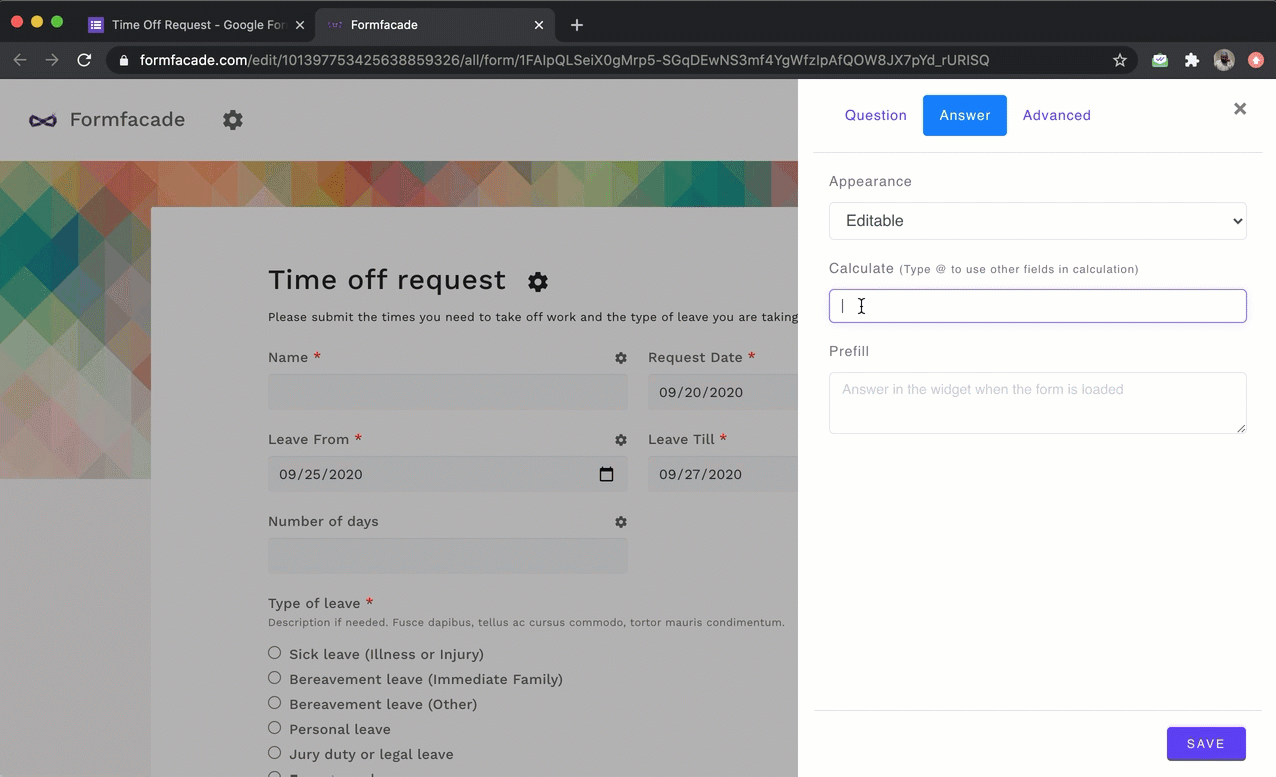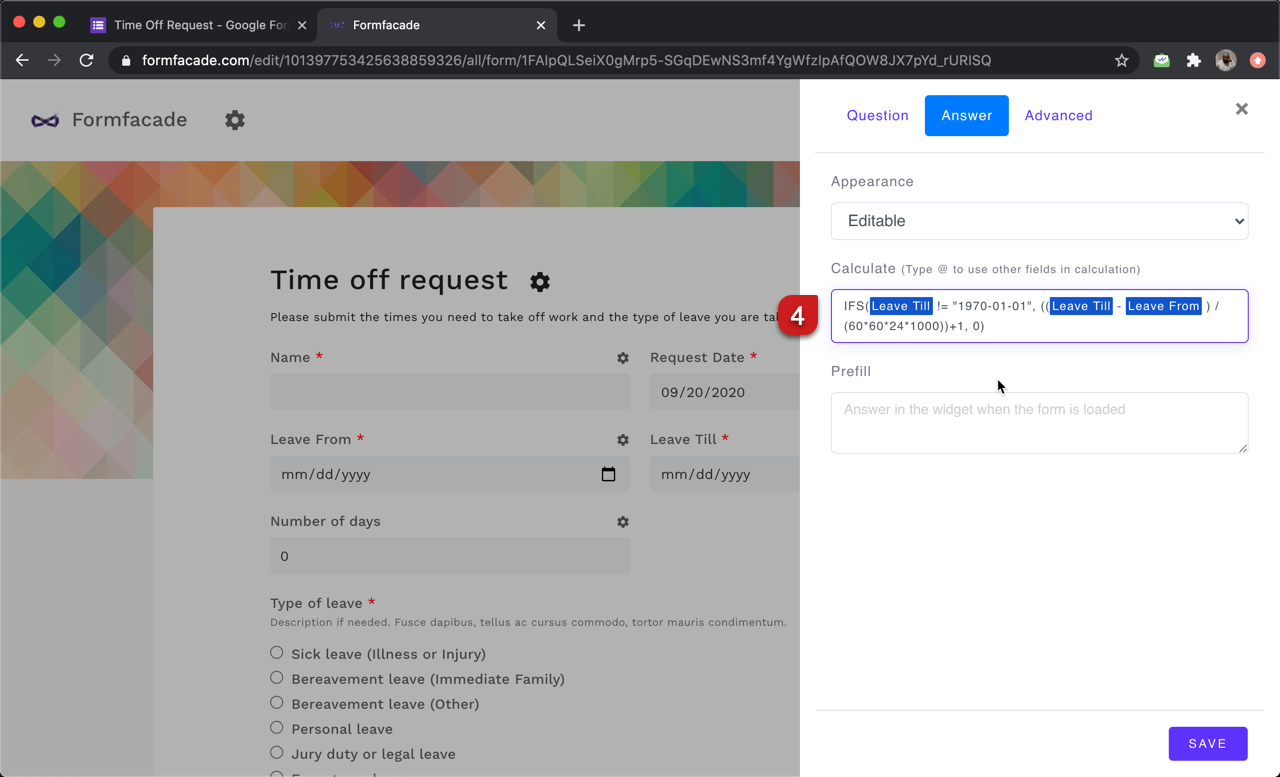# How to calculate the days between two dates?

### You can subtract the start date from end date to calculate the number of days between the dates. By default, the answer will be in milliseconds. You can divide by 24*60*60*1000 to convert it into days.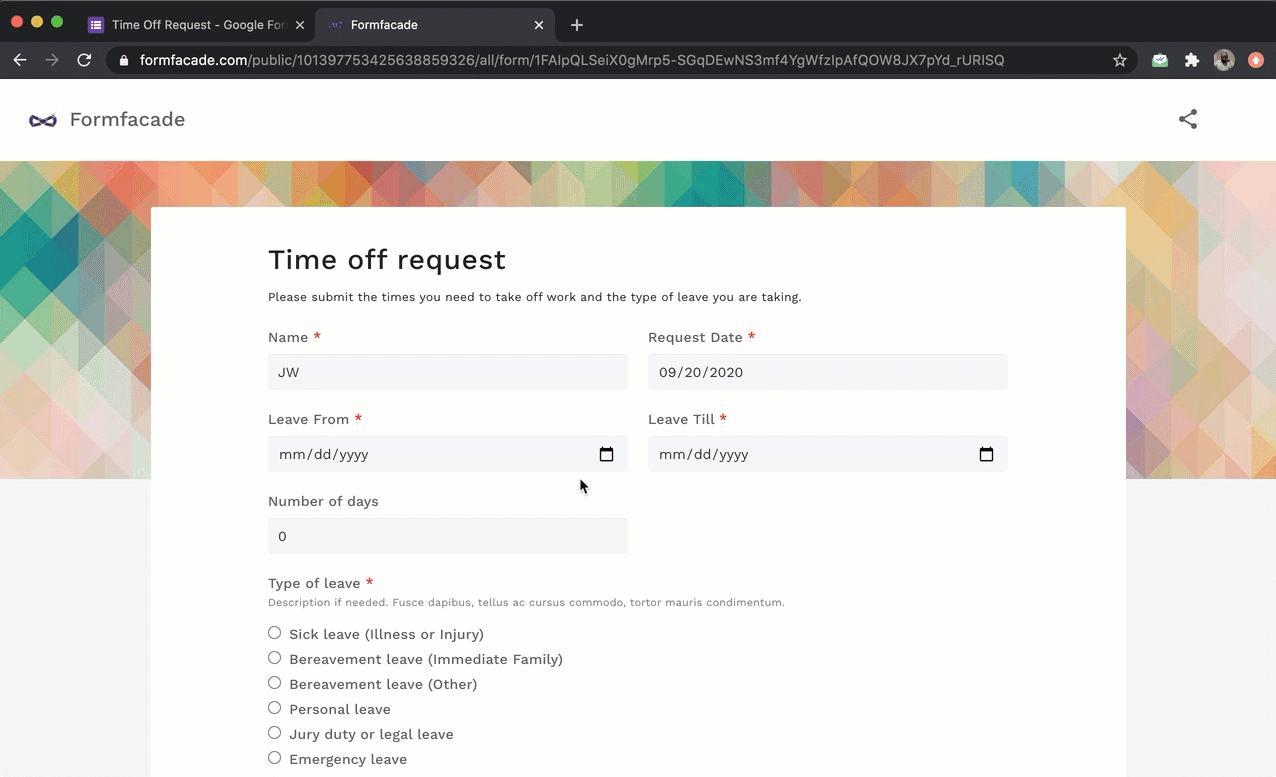Let's take the example of a leave request form. When the user enters the leave start date and leave end date, we can automatically calculate the number of days by writing a formula in the Calculate option for the number of days question.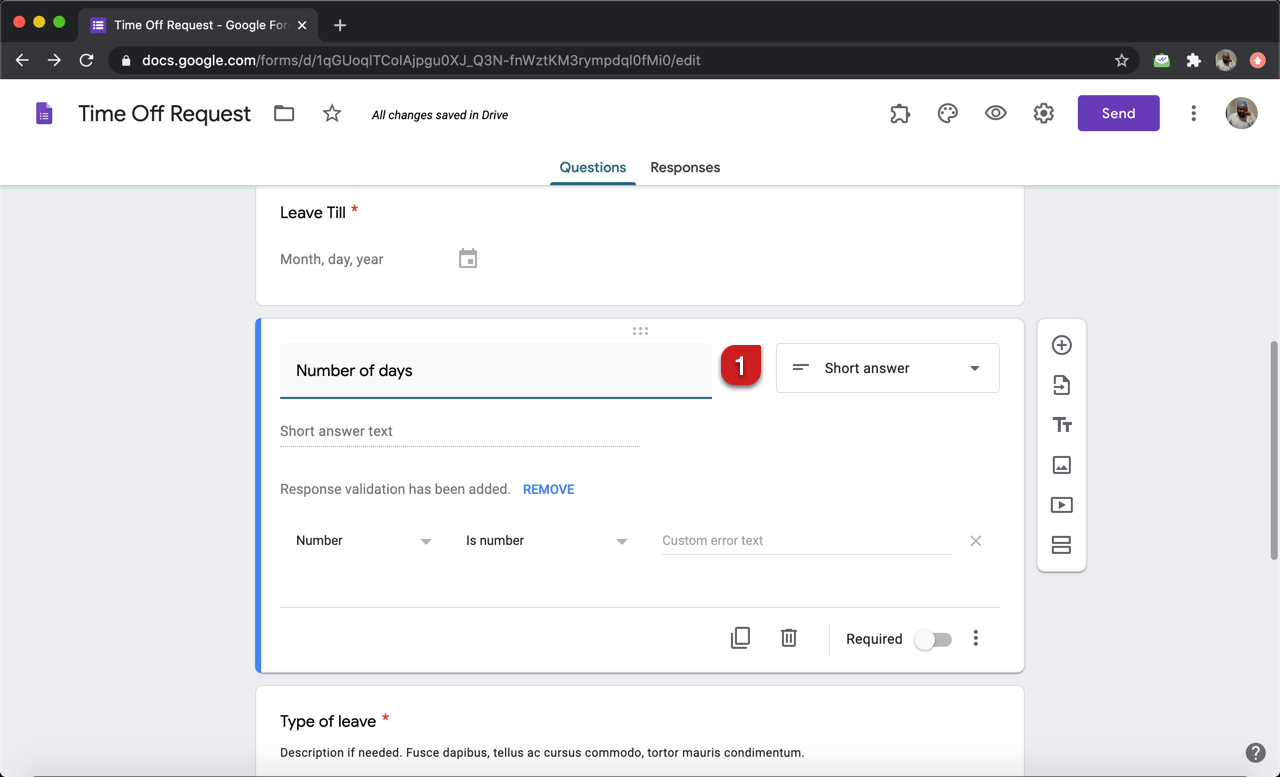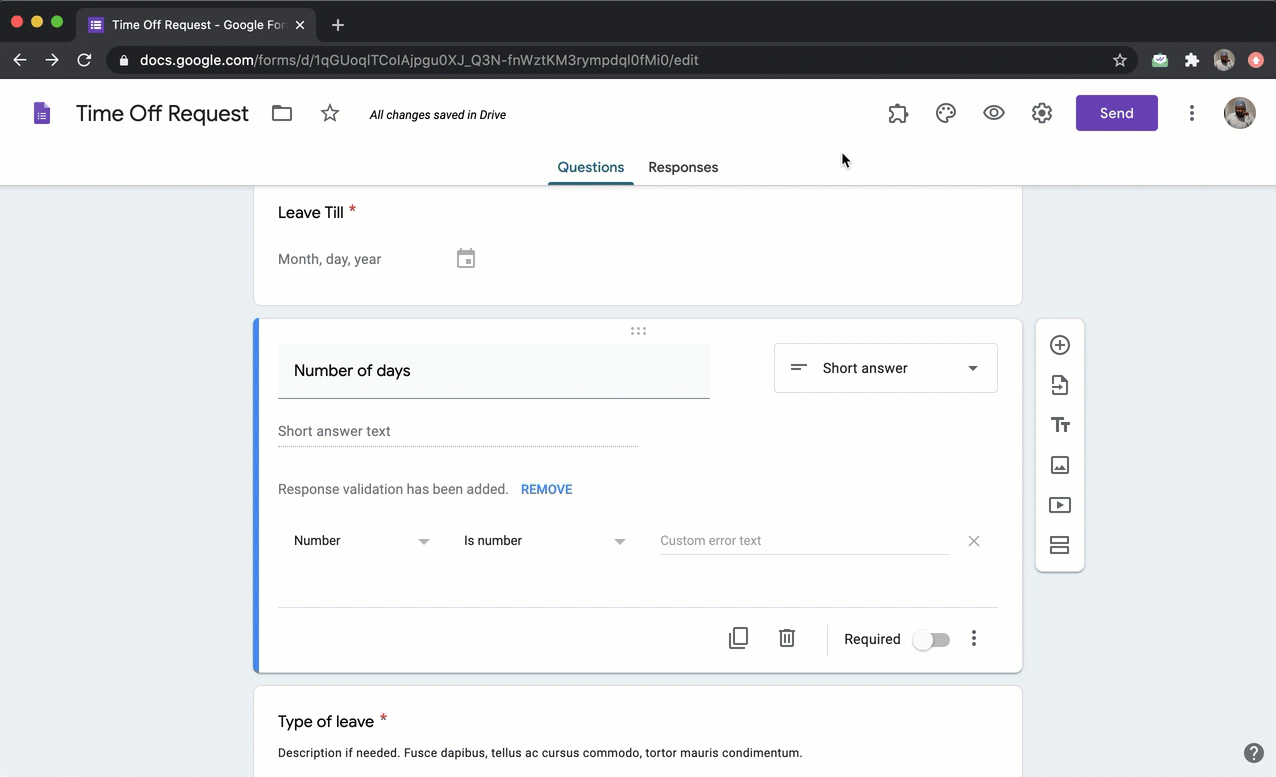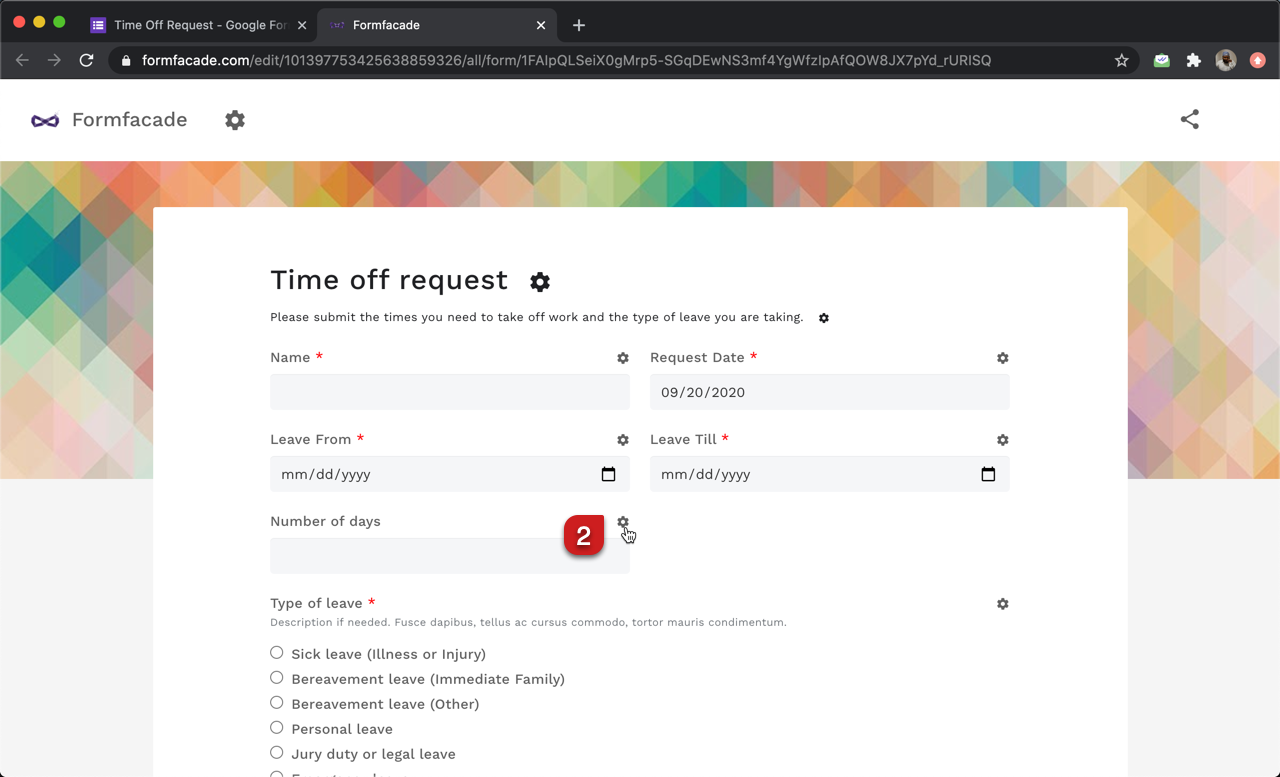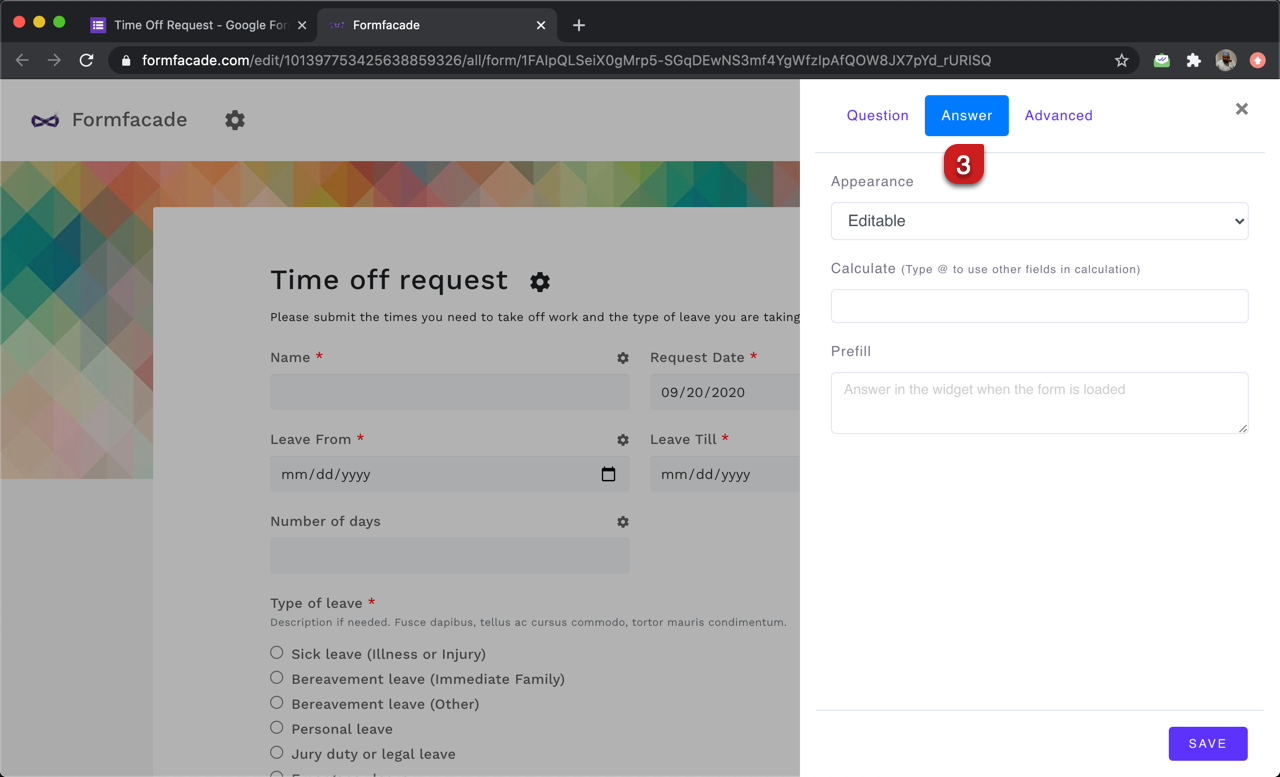#### Number of days between two dates

When you subtract the start date from the end date, the result will be in milliseconds. You can divide the answer by 24 * 60 * 60 * 1000 to convert it into number of days. Please note that you must add 1 to the results to include the start date.

((Leave Till - Leave From) / (24 * 60 * 60 * 1000)) + 1

Divide by 1000 to convert into seconds
Divide by (60 * 1000) to convert into minutes
Divide by (60 * 60 * 1000) to convert into hours
Divide by (24 * 60 * 60 * 1000) to convert into days